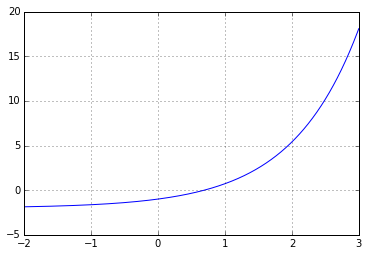# Convergence of Newton's Method¶

In :
import numpy as np
import matplotlib.pyplot as pt

In :
def f(x):
return np.exp(x) - 2

def df(x):
return np.exp(x)

In :
xgrid = np.linspace(-2, 3, 1000)
pt.grid()
pt.plot(xgrid, f(xgrid))

Out:
[<matplotlib.lines.Line2D at 0x7fec13f69048>]What's the true solution of $f(x)=0$?

In :
xtrue = np.log(2)
print(xtrue)
print(f(xtrue))

0.69314718056
0.0


Now let's run Newton's method and keep track of the errors:

In :
errors = []
x = 2


At each iteration, print the current guess and the error.

In :
x = x - f(x)/df(x)
print(x)
errors.append(abs(x-xtrue))
print(errors[-1])

0.69314718056
0.0

In :
for err in errors:
print(err)

0.577523385913
0.13881012318
0.00920340345722
4.22216905669e-05
8.91323015395e-10
0.0

• Do you have a hypothesis about the order of convergence?
In :
# Doubles number of digits each iteration: probably quadratic.


Let's check:

In :
for i in range(len(errors)-1):
print(errors[i+1]/errors[i]**2)

0.416180751055
0.47764604026
0.498469622214
0.499992953401
0.0